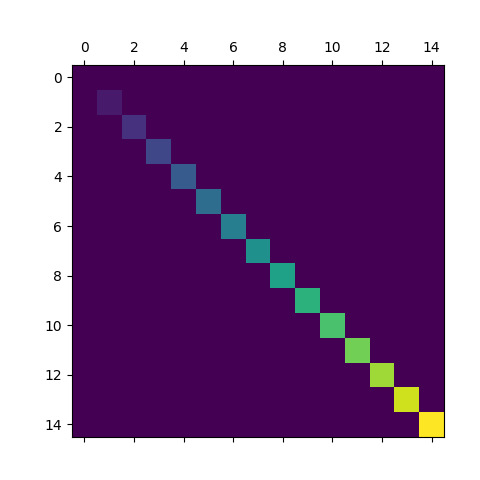# Matshow¶

matshow visualizes a 2D matrix or array as color-coded image.

import matplotlib.pyplot as plt
import numpy as np

# a 2D array with linearly increasing values on the diagonal
a = np.diag(range(15))

plt.matshow(a)

plt.show()